Shape Construction Quiz II, Non Verbal Reasoning

# Shape Construction Quiz II, Non Verbal Reasoning - Police Constable Exams

Test Description

## 25 Questions MCQ Test General Intelligence & Reasoning for Police Exams - Shape Construction Quiz II, Non Verbal Reasoning

Shape Construction Quiz II, Non Verbal Reasoning for Police Constable Exams 2023 is part of General Intelligence & Reasoning for Police Exams preparation. The Shape Construction Quiz II, Non Verbal Reasoning questions and answers have been prepared according to the Police Constable Exams exam syllabus.The Shape Construction Quiz II, Non Verbal Reasoning MCQs are made for Police Constable Exams 2023 Exam. Find important definitions, questions, notes, meanings, examples, exercises, MCQs and online tests for Shape Construction Quiz II, Non Verbal Reasoning below.
Solutions of Shape Construction Quiz II, Non Verbal Reasoning questions in English are available as part of our General Intelligence & Reasoning for Police Exams for Police Constable Exams & Shape Construction Quiz II, Non Verbal Reasoning solutions in Hindi for General Intelligence & Reasoning for Police Exams course. Download more important topics, notes, lectures and mock test series for Police Constable Exams Exam by signing up for free. Attempt Shape Construction Quiz II, Non Verbal Reasoning | 25 questions in 50 minutes | Mock test for Police Constable Exams preparation | Free important questions MCQ to study General Intelligence & Reasoning for Police Exams for Police Constable Exams Exam | Download free PDF with solutions
 1 Crore+ students have signed up on EduRev. Have you?
Shape Construction Quiz II, Non Verbal Reasoning - Question 1

### Directions to Solve In each of the following questions, a set of five alternative figures 1, 2, 3, 4 and 5 followed by a set of four alternatives (A), (B), (C) and (D) is provided. It is required to select the alternative which represents three out of the five alternative figures which when fitted into each other would form a complete square. Question - Select the alternative which represents three out of the five alternative figures which when fitted into each other would form a complete square.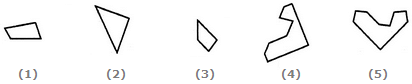Detailed Solution for Shape Construction Quiz II, Non Verbal Reasoning - Question 1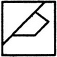Shape Construction Quiz II, Non Verbal Reasoning - Question 2

### Directions to Solve In each of the following questions, a set of five alternative figures 1, 2, 3, 4 and 5 followed by a set of four alternatives (A), (B), (C) and (D) is provided. It is required to select the alternative which represents three out of the five alternative figures which when fitted into each other would form a complete square. Question - Select the alternative which represents three out of the five alternative figures which when fitted into each other would form a complete square.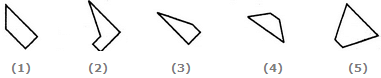Detailed Solution for Shape Construction Quiz II, Non Verbal Reasoning - Question 2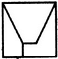Shape Construction Quiz II, Non Verbal Reasoning - Question 3

### Directions to Solve In each of the following questions, a set of five alternative figures 1, 2, 3, 4 and 5 followed by a set of four alternatives (A), (B), (C) and (D) is provided. It is required to select the alternative which represents three out of the five alternative figures which when fitted into each other would form a equilateral triangle. Question - Select the alternative which represents three out of the five alternative figures which when fitted into each other would form a equilateral triangle.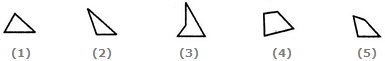Detailed Solution for Shape Construction Quiz II, Non Verbal Reasoning - Question 3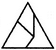Shape Construction Quiz II, Non Verbal Reasoning - Question 4

In each of the following questions, a set of five alternative figures 1, 2, 3, 4 and 5 followed by a set of four alternatives (A), (B), (C) and (D) is provided. It is required to select the alternative which represents three out of the five alternative figures which when fitted into each other would form a equilateral triangle.

Question -

Select the alternative which represents three out of the five alternative figures which when fitted into each other would form a equilateral triangle.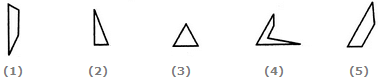Detailed Solution for Shape Construction Quiz II, Non Verbal Reasoning - Question 4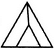Shape Construction Quiz II, Non Verbal Reasoning - Question 5

In each of the following questions, a set of five alternative figures 1, 2, 3, 4 and 5 followed by a set of four alternatives (A), (B), (C) and (D) is provided. It is required to select the alternative which represents three out of the five alternative figures which when fitted into each other would form a equilateral triangle.

Question -

Select the alternative which represents three out of the five alternative figures which when fitted into each other would form a equilateral triangle.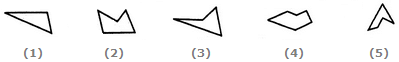Detailed Solution for Shape Construction Quiz II, Non Verbal Reasoning - Question 5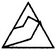Shape Construction Quiz II, Non Verbal Reasoning - Question 6

In each of the following questions, a set of five alternative figures 1, 2, 3, 4 and 5 followed by a set of four alternatives (A), (B), (C) and (D) is provided. It is required to select the alternative which represents three out of the five alternative figures which when fitted into each other would form a equilateral triangle.

Question -

Select the alternative which represents three out of the five alternative figures which when fitted into each other would form a equilateral triangle.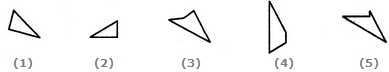Detailed Solution for Shape Construction Quiz II, Non Verbal Reasoning - Question 6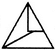Shape Construction Quiz II, Non Verbal Reasoning - Question 7

In each of the following questions, a set of five alternative figures 1, 2, 3, 4 and 5 followed by a set of four alternatives (A), (B), (C) and (D) is provided. It is required to select the alternative which represents three out of the five alternative figures which when fitted into each other would form a equilateral triangle.

Question -

Select the alternative which represents three out of the five alternative figures which when fitted into each other would form a equilateral triangle.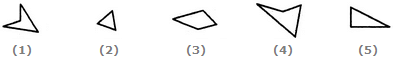Detailed Solution for Shape Construction Quiz II, Non Verbal Reasoning - Question 7Shape Construction Quiz II, Non Verbal Reasoning - Question 8

In each of the following questions, a set of five alternative figures 1, 2, 3, 4 and 5 followed by a set of four alternatives (A), (B), (C) and (D) is provided. It is required to select the alternative which represents three out of the five alternative figures which when fitted into each other would form a equilateral triangle.

Question -

Select the alternative which represents three out of the five alternative figures which when fitted into each other would form a equilateral triangle.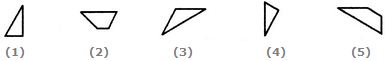Detailed Solution for Shape Construction Quiz II, Non Verbal Reasoning - Question 8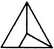Shape Construction Quiz II, Non Verbal Reasoning - Question 9

In each of the following questions, a set of five alternative figures 1, 2, 3, 4 and 5 followed by a set of four alternatives (A), (B), (C) and (D) is provided. It is required to select the alternative which represents three out of the five alternative figures which when fitted into each other would form a equilateral triangle.

Question -

Select the alternative which represents three out of the five alternative figures which when fitted into each other would form a equilateral triangle.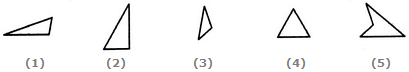Detailed Solution for Shape Construction Quiz II, Non Verbal Reasoning - Question 9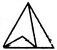Shape Construction Quiz II, Non Verbal Reasoning - Question 10

In each of the following questions, a set of five alternative figures 1, 2, 3, 4 and 5 followed by a set of four alternatives (A), (B), (C) and (D) is provided. It is required to select the alternative which represents three out of the five alternative figures which when fitted into each other would form a equilateral triangle.

Question -

Select the alternative which represents three out of the five alternative figures which when fitted into each other would form a equilateral triangle.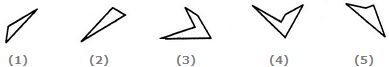Detailed Solution for Shape Construction Quiz II, Non Verbal Reasoning - Question 10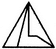Shape Construction Quiz II, Non Verbal Reasoning - Question 11

In each of the following questions, a set of five alternative figures 1, 2, 3, 4 and 5 followed by a set of four alternatives (A), (B), (C) and (D) is provided. It is required to select the alternative which represents three out of the five alternative figures which when fitted into each other would form a equilateral triangle.

Question -

Select the alternative which represents three out of the five alternative figures which when fitted into each other would form a equilateral triangle.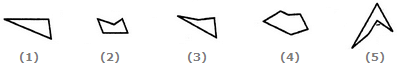Detailed Solution for Shape Construction Quiz II, Non Verbal Reasoning - Question 11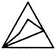Shape Construction Quiz II, Non Verbal Reasoning - Question 12

In each of the following questions, a set of five alternative figures 1, 2, 3, 4 and 5 followed by a set of four alternatives (A), (B), (C) and (D) is provided. It is required to select the alternative which represents three out of the five alternative figures which when fitted into each other would form a equilateral triangle.

Question -

Select the alternative which represents three out of the five alternative figures which when fitted into each other would form a equilateral triangle.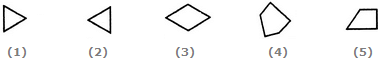Detailed Solution for Shape Construction Quiz II, Non Verbal Reasoning - Question 12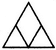Shape Construction Quiz II, Non Verbal Reasoning - Question 13

In each of the following questions, a key figure marked (X) is given, followed by four other alternative figures marked (1), (2), (3) and (4). It is required to select one figure from the alternatives, which fits exactly into figure (X) to form a complete square.

Question -

Select a figure from the given four alternatives which fits exactly into Figure-X to form a complete square.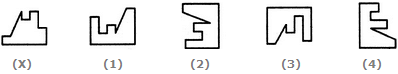Detailed Solution for Shape Construction Quiz II, Non Verbal Reasoning - Question 13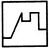Shape Construction Quiz II, Non Verbal Reasoning - Question 14

In each of the following questions, a key figure marked (X) is given, followed by four other alternative figures marked (1), (2), (3) and (4). It is required to select one figure from the alternatives, which fits exactly into figure (X) to form a complete square.

Question -

Select a figure from the given four alternatives which fits exactly into Figure-X to form a complete square.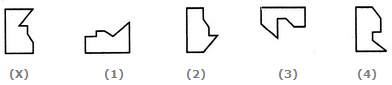Detailed Solution for Shape Construction Quiz II, Non Verbal Reasoning - Question 14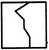Shape Construction Quiz II, Non Verbal Reasoning - Question 15

In each of the following questions, a key figure marked (X) is given, followed by four other alternative figures marked (1), (2), (3) and (4). It is required to select one figure from the alternatives, which fits exactly into figure (X) to form a complete square.

Question -

Select a figure from the given four alternatives which fits exactly into Figure-X to form a complete square.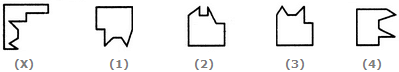Detailed Solution for Shape Construction Quiz II, Non Verbal Reasoning - Question 15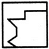Shape Construction Quiz II, Non Verbal Reasoning - Question 16

In each of the following questions, a key figure marked (X) is given, followed by four other alternative figures marked (1), (2), (3) and (4). It is required to select one figure from the alternatives, which fits exactly into figure (X) to form a complete square.

Question -

Select a figure from the given four alternatives which fits exactly into Figure-X to form a complete square.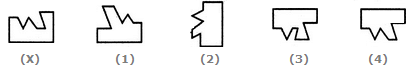Detailed Solution for Shape Construction Quiz II, Non Verbal Reasoning - Question 16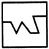Shape Construction Quiz II, Non Verbal Reasoning - Question 17

In each of the following questions, a key figure marked (X) is given, followed by four other alternative figures marked (1), (2), (3) and (4). It is required to select one figure from the alternatives, which fits exactly into figure (X) to form a complete square.

Question -

Select a figure from the given four alternatives which fits exactly into Figure-X to form a complete square.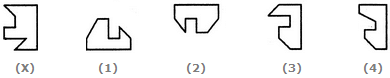Detailed Solution for Shape Construction Quiz II, Non Verbal Reasoning - Question 17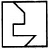Shape Construction Quiz II, Non Verbal Reasoning - Question 18

In each of the following questions, a key figure marked (X) is given, followed by four other alternative figures marked (1), (2), (3) and (4). It is required to select one figure from the alternatives, which fits exactly into figure (X) to form a complete square.

Question -

Select a figure from the given four alternatives which fits exactly into Figure-X to form a complete square.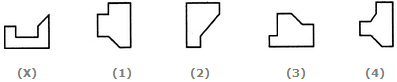Detailed Solution for Shape Construction Quiz II, Non Verbal Reasoning - Question 18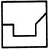Shape Construction Quiz II, Non Verbal Reasoning - Question 19

In each of the following questions, a key figure marked (X) is given, followed by four other alternative figures marked (1), (2), (3) and (4). It is required to select one figure from the alternatives, which fits exactly into figure (X) to form a complete square.

Question -

Select a figure from the given four alternatives which fits exactly into Figure-X to form a complete square.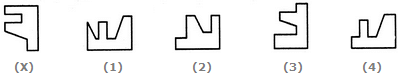Detailed Solution for Shape Construction Quiz II, Non Verbal Reasoning - Question 19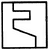Shape Construction Quiz II, Non Verbal Reasoning - Question 20

In each of the following questions, a key figure marked (X) is given, followed by four other alternative figures marked (1), (2), (3) and (4). It is required to select one figure from the alternatives, which fits exactly into figure (X) to form a complete square.

Question -

Select a figure from the given four alternatives which fits exactly into Figure-X to form a complete square.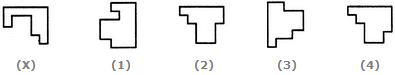Detailed Solution for Shape Construction Quiz II, Non Verbal Reasoning - Question 20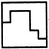Shape Construction Quiz II, Non Verbal Reasoning - Question 21

In each of the following questions, a key figure marked (X) is given, followed by four other alternative figures marked (1), (2), (3) and (4). It is required to select one figure from the alternatives, which fits exactly into figure (X) to form a complete square.

Question -

Select a figure from the given four alternatives which fits exactly into Figure-X to form a complete square.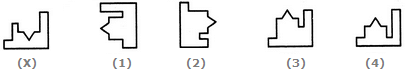Detailed Solution for Shape Construction Quiz II, Non Verbal Reasoning - Question 21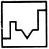Shape Construction Quiz II, Non Verbal Reasoning - Question 22

In each of the following questions, a key figure marked (X) is given, followed by four other alternative figures marked (1), (2), (3) and (4). It is required to select one figure from the alternatives, which fits exactly into figure (X) to form a complete square.

Question -

Select a figure from the given four alternatives which fits exactly into Figure-X to form a complete square.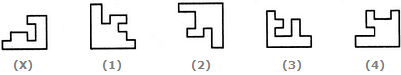Detailed Solution for Shape Construction Quiz II, Non Verbal Reasoning - Question 22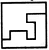Shape Construction Quiz II, Non Verbal Reasoning - Question 23

In each of the following questions, a key figure marked (X) is given, followed by four other alternative figures marked (1), (2), (3) and (4). It is required to select one figure from the alternatives, which fits exactly into figure (X) to form a complete square.

Question -

Select a figure from the given four alternatives which fits exactly into Figure-X to form a complete square.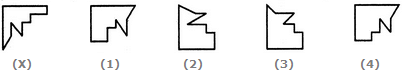Detailed Solution for Shape Construction Quiz II, Non Verbal Reasoning - Question 23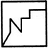Shape Construction Quiz II, Non Verbal Reasoning - Question 24

In each of the following questions, a key figure marked (X) is given, followed by four other alternative figures marked (1), (2), (3) and (4). It is required to select one figure from the alternatives, which fits exactly into figure (X) to form a complete square.

Question -

Select a figure from the given four alternatives which fits exactly into Figure-X to form a complete square.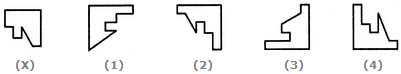Detailed Solution for Shape Construction Quiz II, Non Verbal Reasoning - Question 24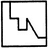Shape Construction Quiz II, Non Verbal Reasoning - Question 25

In each of the following questions, a key figure marked (X) is given, followed by four other alternative figures marked (1), (2), (3) and (4). It is required to select one figure from the alternatives, which fits exactly into figure (X) to form a complete square.

Question -

Select a figure from the given four alternatives which fits exactly into Figure-X to form a complete square.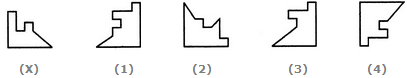Detailed Solution for Shape Construction Quiz II, Non Verbal Reasoning - Question 25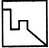## General Intelligence & Reasoning for Police Exams

83 videos|44 docs|96 tests
Information about Shape Construction Quiz II, Non Verbal Reasoning Page
In this test you can find the Exam questions for Shape Construction Quiz II, Non Verbal Reasoning solved & explained in the simplest way possible. Besides giving Questions and answers for Shape Construction Quiz II, Non Verbal Reasoning, EduRev gives you an ample number of Online tests for practice

## General Intelligence & Reasoning for Police Exams

83 videos|44 docs|96 tests